# Time Series Analysis/Forecasting and Univariate ARIMA Models¶

In :
import os
import sys
import warnings
from datetime import date
import pandas as pd
import numpy as np
from numpy.linalg import LinAlgError

import statsmodels.tsa.api as tsa
from statsmodels.graphics.tsaplots import plot_acf, acf, plot_pacf, pacf
from statsmodels.tsa.stattools import acf, q_stat, adfuller
import statsmodels.api as sm
from scipy.stats import probplot, moment
from sklearn.metrics import mean_squared_error

import seaborn as sns
import matplotlib.pyplot as plt
import matplotlib as mpl

In :
%matplotlib inline
warnings.filterwarnings('ignore')
sns.set(style="darkgrid", color_codes=True)

In :
def plot_correlogram(x, lags=None, title=None):
lags = min(10, int(len(x)/5)) if lags is None else lags
fig, axes = plt.subplots(nrows=2, ncols=2, figsize=(13, 8))
x.plot(ax=axes)
q_p = np.max(q_stat(acf(x, nlags=lags), len(x)))
axes.text(x=.02, y=.85, s=stats, transform=axes.transAxes)
probplot(x, plot=axes)
mean, var, skew, kurtosis = moment(x, moment=[1, 2, 3, 4])
s = f'Mean: {mean:>12.2f}\nSD: {np.sqrt(var):>16.2f}\nSkew: {skew:12.2f}\nKurtosis:{kurtosis:9.2f}'
axes.text(x=.02, y=.75, s=s, transform=axes.transAxes)
plot_acf(x=x, lags=lags, zero=False, ax=axes)
plot_pacf(x, lags=lags, zero=False, ax=axes)
axes.set_xlabel('Lag')
axes.set_xlabel('Lag')
fig.suptitle(title, fontsize=20)
fig.tight_layout()


Load monthly Industrial Production and daily NASDAQ stock market index:

In :
industrial_production = web.DataReader('IPGMFN', 'fred', '1988', '2020-02').squeeze().dropna()
nasdaq = web.DataReader('NASDAQCOM', 'fred', '1990', '2020-03-31').squeeze().dropna()

In :
industrial_production

Out:
DATE
1988-01-01     56.7982
1988-02-01     58.0084
1988-03-01     58.6871
1988-04-01     58.9176
1988-05-01     59.0147
...
2019-10-01    106.2767
2019-11-01    105.7610
2019-12-01    104.4315
2020-01-01    103.4507
2020-02-01    105.4971
Name: IPGMFN, Length: 386, dtype: float64
In :
nasdaq

Out:
DATE
1990-01-02     459.330
1990-01-03     460.900
1990-01-04     459.390
1990-01-05     458.220
1990-01-08     458.710
...
2020-03-25    7384.297
2020-03-26    7797.535
2020-03-27    7502.379
2020-03-30    7774.152
2020-03-31    7700.098
Name: NASDAQCOM, Length: 7622, dtype: float64

## Plot Series¶

In :
fig, ax = plt.subplots(2,1,figsize=(13, 8))

ax.plot(nasdaq, color='b')
ax.plot(industrial_production, color='r')

ax.set_xlabel('Date')
ax.set_xlabel('Date')

ax.set_title('NASDAQ', color='b')
ax.set_title('Industrial Production', color='r')

ax.set_ylabel('NASDAQ Close ()', color='b') ax.set_ylabel('Production Count', color='r') ax.grid(False) ax.grid(False) plt.tight_layout() plt.show()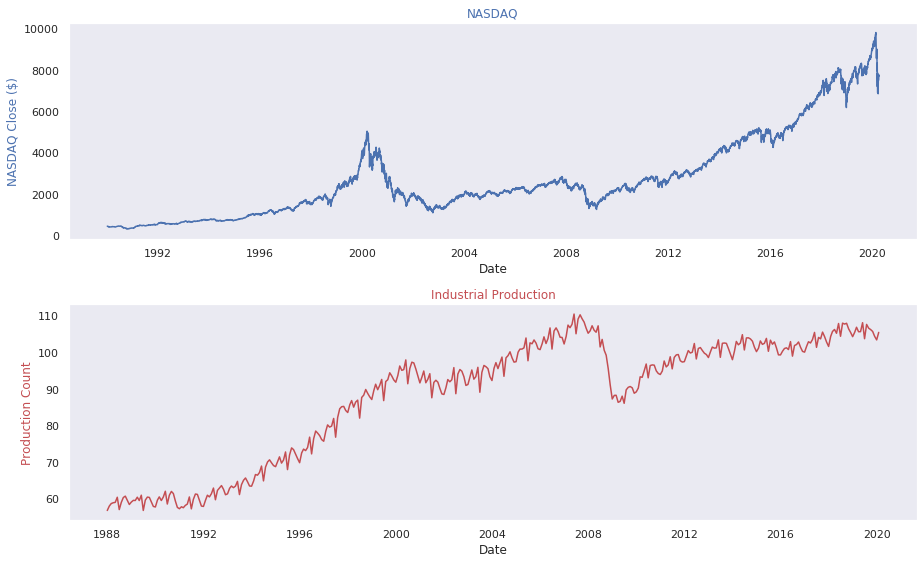## Additive Decomposition¶ Time series data typically contains a mix of various patterns that can be decomposed into several components, each representing an underlying pattern category. In particular, time series often consist of the systematic components trend, seasonality and cycles, and unsystematic noise(white noise). These components can be combined in an additive, linear model, in particular when fluctuations do not depend on the level of the series, or in a non-linear, multiplicative model. These components can be split up automatically. statsmodels includes a simple method to split the time series into a trend, seasonal, and residual component using moving averages. We can apply it to monthly data on industrial manufacturing production with both a strong trend and seasonality component, as follows: In : components = tsa.seasonal_decompose(industrial_production, model='additive')  In : ts = (industrial_production.to_frame('Original') .assign(Trend=components.trend) .assign(Seasonality=components.seasonal) .assign(Residual=components.resid)) ts.plot(subplots=True, figsize=(13, 10)) plt.show()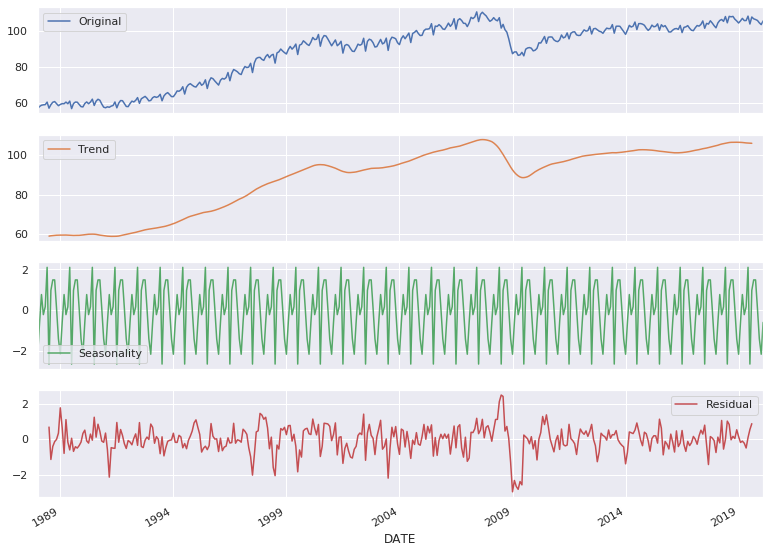## Time Series Stationarity¶ The statistical properties, such as the mean, variance, or autocorrelation, of a stationary time series don't change over time. Hence, stationarity implies that a time series does not have any trend or seasonal effects and that descriptive statistics, such as the mean or the standard deviation, when computed for different rolling windows, remain constant or do not change much over time. It reverts to its mean, and the deviations have constant amplitude, while short-term movements always look fairly similar. Strict stationarity requires the joint distribution of any subset of time series observations to be independent of time with respect to all moments. So, in addition to the mean and variance, higher moments such as skew and kurtosis, also need to be constant, irrespective of the lag between different observations. In most applications, we limit stationarity to first and second moments so that the time series is covariance stationary with constant mean, variance, and autocorrelation. Note that we specifically allow for dependence between observations at different lags, just like we want the input data for linear regression to be correlated with the outcome. Stationarity implies that these relationships are stable, which facilitates prediction as the model can focus on learning systematic patterns that take place within stable statistical properties. To satisfy the stationarity assumption of linear time series models, we need to transform the original time series, often in several steps. Common transformations include: • Taking the (natural) logarithm to convert an exponential growth pattern into a linear trend and stabilize the variance. • Deflation implies dividing a time series by another series that causes trending behavior, for example dividing a nominal series by a price index to convert it into a real measure. • Differencing a time-series meaning for each value in the time-series we subtract its previous value.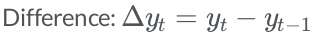Other Transformations: • Take the square root: • np.sqrt(df) • Take the proportional change: • df.shift(1)/df ### Test for stationarity¶ The augmented Dicky-Fuller test • Tests for trend non-stationarity • Null hypothesis is time series is non-stationary In : adf = adfuller(industrial_production) print(f'Test Statistic: {adf}') print(f'\nP-Value: {adf}') print(f'\nCritical Values: {adf}')  Test Statistic: -1.5867425644697801 P-Value: 0.4902496718328939 Critical Values: {'1%': -3.448196541708585, '5%': -2.869404683789669, '10%': -2.5709597356805545}  • 0th element is test statistic (-1.59) • More negative means more likely to be stationary • 1st element is p-value: (0.49) • If p-value is small → reject null hypothesis. Reject non-stationary. • 4th element is the critical test statistics ### Log Transformation¶ Check for zero values In : (nasdaq == 0).any(), (industrial_production==0).any()  Out: (False, False) In : nasdaq_log = np.log(nasdaq) industrial_production_log = np.log(industrial_production)  ### Differencing¶ In many cases, de-trending is not sufficient to make the series stationary. Instead, we need to transform the original data into a series of period-to-period and/or season-to-season differences. Note that when such differencing is applied to a log-transformed series, the results represent instantaneous growth rates or returns in a financial context. If a univariate series becomes stationary after differencing d times, it is said to be integrated of the order of d, or simply integrated if d=1. This behavior is due to so-called unit roots. • What is “Unit Root”? • A unit root (also called a unit root process or a difference stationary process) is a stochastic trend in a time series, sometimes called a “random walk with drift”; If a time series has a unit root, it shows a systematic pattern that is unpredictable. Differencing of log series produces instantaneous returns. In : nasdaq_log_diff = nasdaq_log.diff().dropna() # seasonal differencing of 12 = yoy instantanteous returns industrial_production_log_diff = industrial_production_log.diff(12).dropna()  ### Plot Series¶ The following chart shows time series for the NASDAQ stock index and industrial production for the 30 years through 2017 in original form, as well as the transformed versions after applying the logarithm and subsequently applying first and seasonal differences (at lag 12), respectively. The charts also display the ADF p-value, which allows us to reject the hypothesis of unit-root non-stationarity after all transformations in both cases: In : fig, axes = plt.subplots(nrows=3, ncols=2, figsize=(13,7)) nasdaq.plot(ax=axes, title='NASDAQ Composite Index') axes.text(x=.03, y=.85, s=f'ADF: {tsa.adfuller(nasdaq.dropna()):.4f}', transform=axes.transAxes) axes.set_ylabel('Index') nasdaq_log.plot(ax=axes, sharex=axes) axes.text(x=.03, y=.85, s=f'ADFl: {tsa.adfuller(nasdaq_log.dropna()):.4f}', transform=axes.transAxes) axes.set_ylabel('Log') nasdaq_log_diff.plot(ax=axes, sharex=axes) axes.text(x=.03, y=.85, s=f'ADF: {tsa.adfuller(nasdaq_log_diff.dropna()):.4f}', transform=axes.transAxes) axes.set_ylabel('Log, Diff') industrial_production.plot(ax=axes, title='Industrial Production: Manufacturing', color='r') axes.text(x=.03, y=.85, s=f'ADF: {tsa.adfuller(industrial_production):.4f}', transform=axes.transAxes) axes.set_ylabel('Index', color='r') industrial_production_log.plot(ax=axes, sharex=axes, color='r') axes.text(x=.03, y=.85, s=f'ADF: {tsa.adfuller(industrial_production_log.dropna()):.4f}', transform=axes.transAxes) axes.set_ylabel('Log', color='r') industrial_production_log_diff.plot(ax=axes, sharex=axes, color='r') axes.text(x=.83, y=.85, s=f'ADF: {tsa.adfuller(industrial_production_log_diff.dropna()):.4f}', transform=axes.transAxes) axes.set_ylabel('Log, Seasonal Diff', color='r') fig.tight_layout() fig.align_ylabels(axes);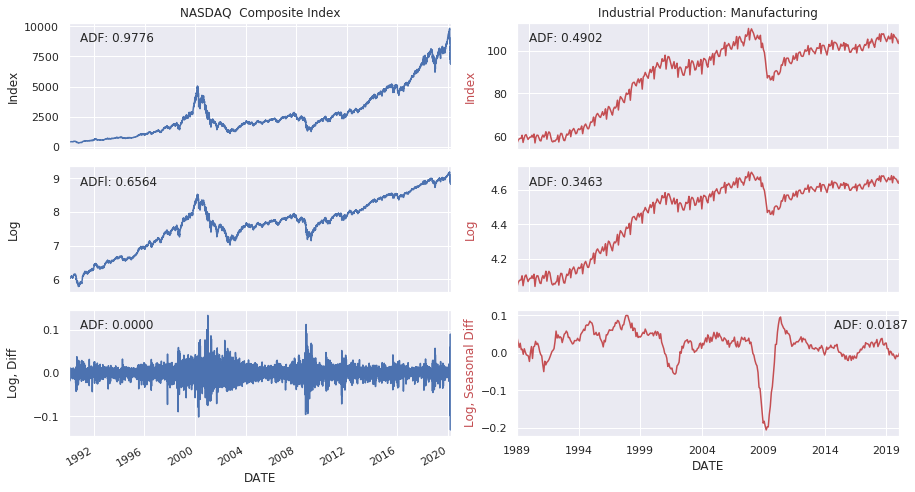## Correlogram¶ Autocorrelation (also called serial correlation) adapts the concept of correlation to the time series context: just as the correlation coefficient measures the strength of a linear relationship between two variables, the autocorrelation coefficient,\rho_k$, measures the extent of a linear relationship between time series values separated by a given lag,$k\$.

Hence, we can calculate one autocorrelation coefficient for each of the T-1 lags in a time series; T is the length of the series. The autocorrelation function (ACF) computes the correlation coefficients as a function of the lags.

The autocorrelation for a lag larger than 1 (that is, between observations more than one time step apart) reflects both the direct correlation between these observations and the indirect influence of the intervening data points.

The partial autocorrelation function (PACF) removes this influence and only measures the linear dependence between data points at the given lag distance. The (PACF) provides all the correlations that result once the effects of correlation at shorter lags have been removed.

A correlogram is simply a plot of the ACF or PACF for sequential lags, k=0,1,...,n. It allows us to inspect the correlation structure across lags at one glance. The main usage of correlograms is to detect any autocorrelation after the removal of the effects of trend or seasonality. Both the ACF and the PACF are key diagnostic tools for the design of linear time series models.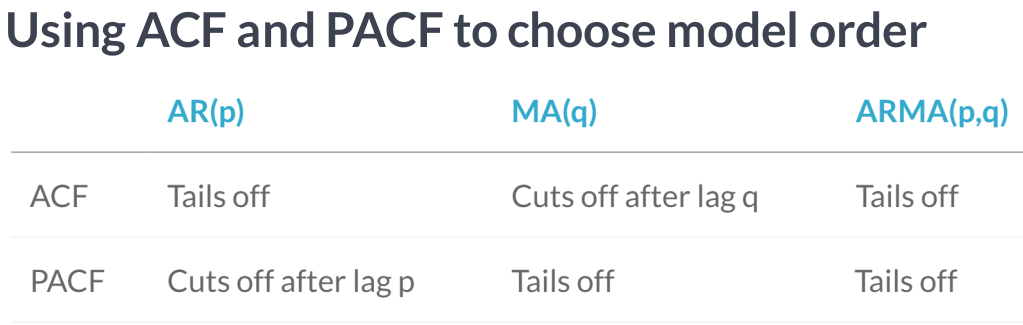### NASDAQ (log, diff)¶

We can further analyze the relevant time series characteristics for the transformed series using a Q-Q plot that compares the quantiles of the distribution of the time series observation to the quantiles of the normal distribution and the correlograms based on the ACF and PACF.

For the NASDAQ plot, we notice that while there is no trend, the variance is not constant but rather shows clustered spikes around periods of market turmoil in the late 1980s, 2001, and 2008. The Q-Q plot highlights the fat tails of the distribution with extreme values more frequent than the normal distribution would suggest. The ACF and the PACF show similar patterns with autocorrelation at several lags appearing significant:

In :
plot_correlogram(nasdaq_log_diff, lags=100, title='NASDAQ Composite (Log, Diff)')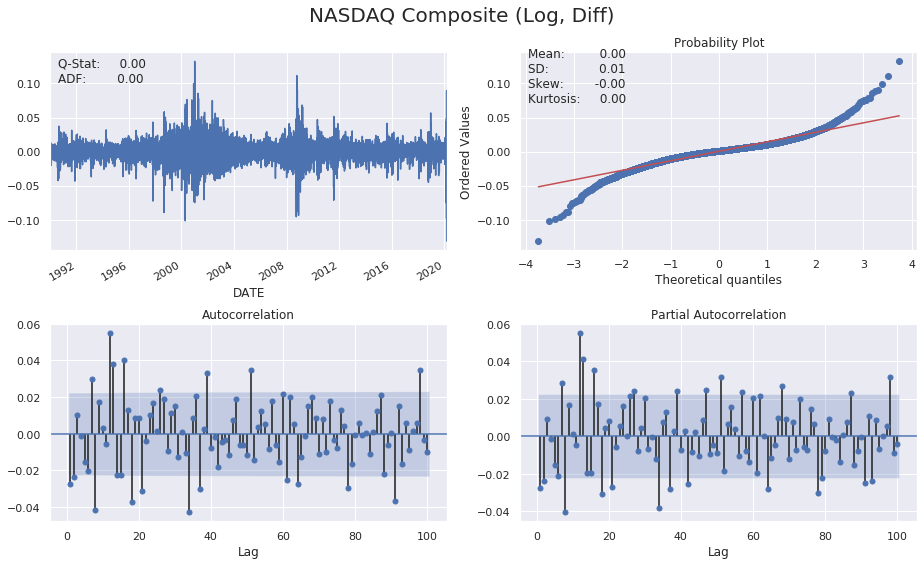### Industrial Production (log, seasonl diff)¶

For the monthly time series on industrial manufacturing production, we notice a large negative outlier following the 2008 crisis as well as the corresponding skew in the Q-Q plot. The autocorrelation is much higher than for the NASDAQ returns and declines smoothly. The PACF shows distinct positive autocorrelation patterns at lag 1 and 13, and significant negative coefficients at lags 3 and 4:

In :
plot_correlogram(industrial_production_log_diff, title='Industrial Production (Seasonal Diff)')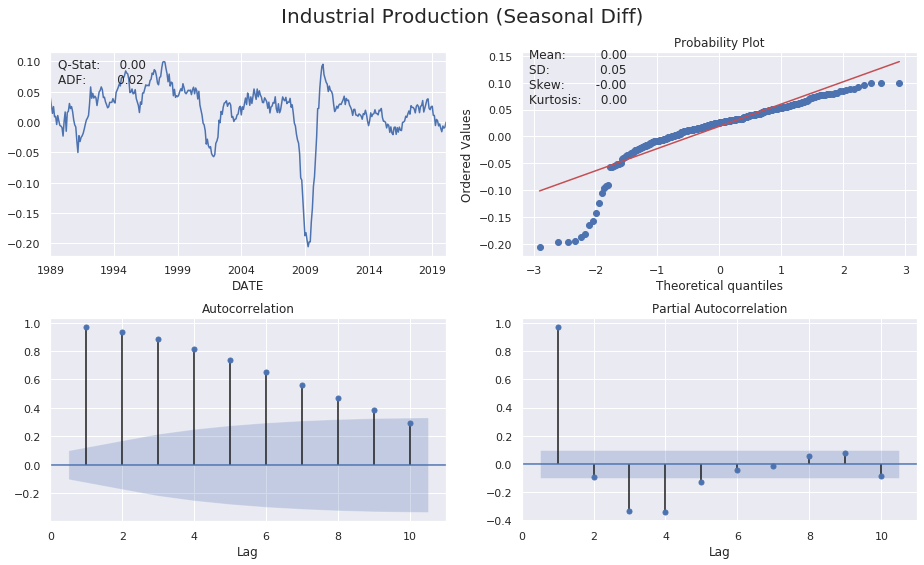## Univariate Time Series Models¶

### Autoregressive (AR) Model¶

Multiple linear-regression models expressed the variable of interest as a linear combination of predictors or input variables. Univariate time series models relate the value of the time series at the point in time of interest to a linear combination of lagged values of the series and possibly past disturbance terms. While exponential smoothing models are based on a description of the trend and seasonality in the data, ARIMA models aim to describe the autocorrelations in the data. ARIMA(p, d, q).

• p - number of autoregressive lags
• d - order of differencing
• q - number of moving average lags

Models require stationarity and leverage two building blocks:

• Autoregressive (AR) terms consisting of p-lagged values of the time series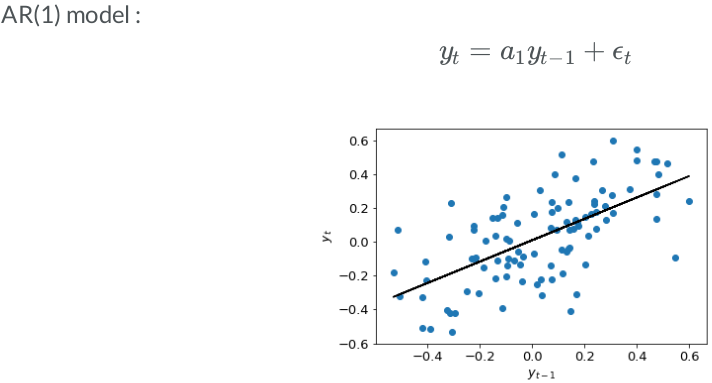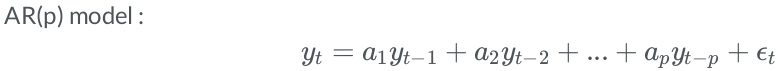• Moving average (MA) terms that contain q-lagged disturbances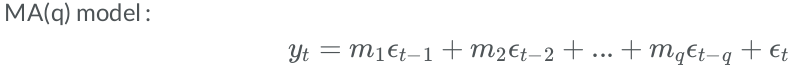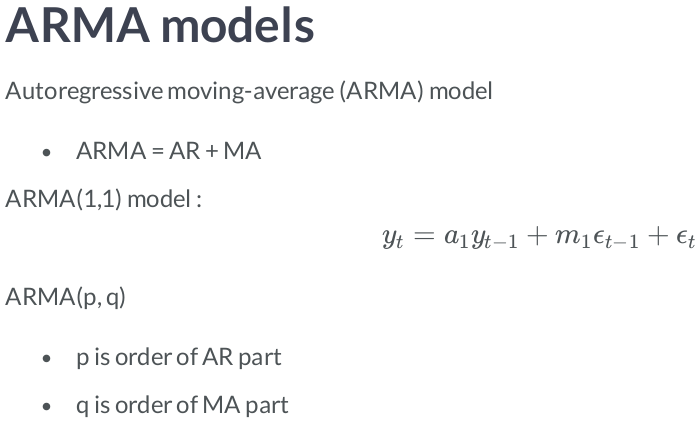### ARMA vs ARIMA¶

The ARMA model of the undifferenced series produces the same result as the ARIMA model of the differenced series.

In :
model1 = tsa.ARMA(endog=nasdaq_log_diff, order=(2,2)).fit()
model2 = tsa.ARIMA(endog=nasdaq_log, order=(2,1,2)).fit()

In :
model1.params.sort_index() == model2.params.sort_index().values

Out:
ar.L1.NASDAQCOM    True
ar.L2.NASDAQCOM    True
const              True
ma.L1.NASDAQCOM    True
ma.L2.NASDAQCOM    True
dtype: bool

### Seasonal differencing vs SARIMAX¶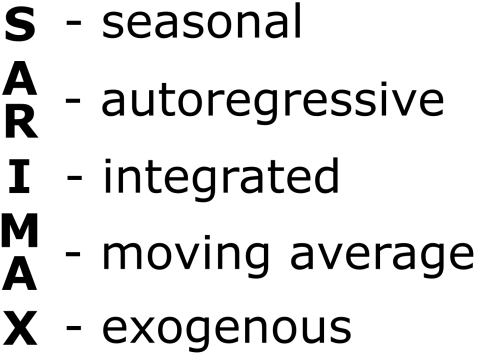Seasonal data

• Has predictable and repeated patterns
• Repeats after any amount of time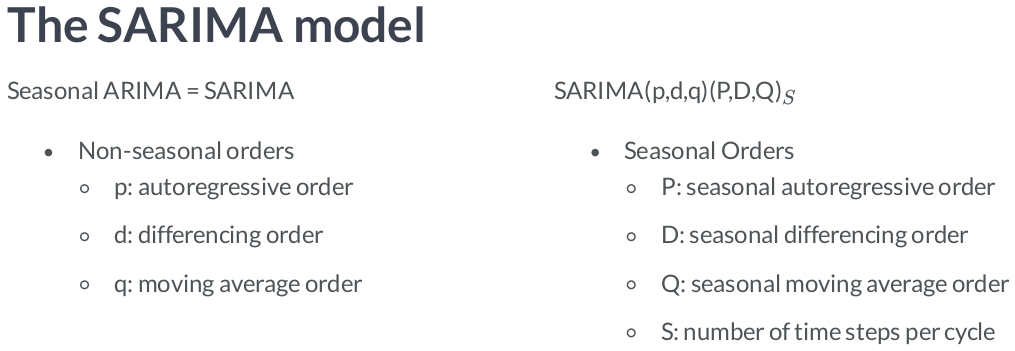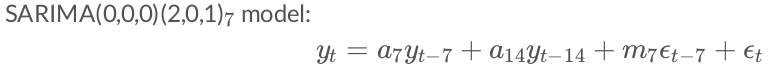Seasonal differencing has same effect as using SARIMAX with seasonal order (0,1,0,12).

In :
model1 = tsa.statespace.SARIMAX(industrial_production_log, order=(2,0,2), seasonal_order=(0,1,0,12)).fit()
model2 = tsa.statespace.SARIMAX(industrial_production_log_diff, order=(2,0,2), seasonal_order=(0,0,0,12)).fit()

In :
model1.params.to_frame('SARIMAX').join(model2.params.to_frame('diff'))

Out:
SARIMAX diff
ar.L1 1.772617 1.810456
ar.L2 -0.793977 -0.832465
ma.L1 -0.853340 -0.915161
ma.L2 0.289099 0.334137
sigma2 0.000100 0.000099

## Finding the optimal ARMA lags¶

### Run candidate models¶

We iterate over various (p, q) lag combinations and collect diagnostic statistics to compare the result.

In :
train_size = 120 # 10 years of training data
test_results = {}
y_true = industrial_production_log_diff.iloc[train_size:]
for p in range(5):
for q in range(5):
aic, bic = [], []
if p == 0 and q == 0:
continue
print(f'p:{p}, q:{q}')
convergence_error = stationarity_error = 0
y_pred = []
for T in range(train_size, len(industrial_production_log_diff)):
train_set = industrial_production_log_diff.iloc[T-train_size:T]
try:
model = tsa.ARMA(endog=train_set, order=(p, q)).fit()
except LinAlgError:
convergence_error += 1
except ValueError:
stationarity_error += 1

forecast, _, _ = model.forecast(steps=1)
y_pred.append(forecast)
aic.append(model.aic)
bic.append(model.bic)

result = (pd.DataFrame({'y_true': y_true, 'y_pred': y_pred})
.replace(np.inf, np.nan)
.dropna())

rmse = np.sqrt(mean_squared_error(
y_true=result.y_true, y_pred=result.y_pred))

test_results[(p, q)] = [rmse,
np.mean(aic),
np.mean(bic),
convergence_error,
stationarity_error]

p:0, q:1
p:0, q:2
p:0, q:3
p:0, q:4
p:1, q:0
p:1, q:1
p:1, q:2
p:1, q:3
p:1, q:4
p:2, q:0
p:2, q:1
p:2, q:2
p:2, q:3
p:2, q:4
p:3, q:0
p:3, q:1
p:3, q:2
p:3, q:3
p:3, q:4
p:4, q:0
p:4, q:1
p:4, q:2
p:4, q:3
p:4, q:4


## AIC and BIC¶

AIC = Akaike information criterion

• A Lower AIC indicates a better model
• AIC likes to choose simpler models with a lower order

BIC = Bayesian information criterion

• Very similar to AIC
• A Lower BIC indicates a better model

AIC vs BIC

• BIC favors simpler models than AIC
• AIC is better at choosing predictive models
• BIC is better at choosing good explanatory model
In :
test_results = pd.DataFrame(test_results).T
test_results.columns = ['RMSE', 'AIC', 'BIC', 'convergence', 'stationarity']
test_results.index.names = ['p', 'q']
test_results.info()

<class 'pandas.core.frame.DataFrame'>
MultiIndex: 24 entries, (0, 1) to (4, 4)
Data columns (total 5 columns):
RMSE            24 non-null float64
AIC             24 non-null float64
BIC             24 non-null float64
convergence     24 non-null float64
stationarity    24 non-null float64
dtypes: float64(5)
memory usage: 1.2 KB

In :
# Sort by AIC

Out:
RMSE AIC BIC convergence stationarity
p q
3 4 0.040955 -762.945922 -737.858496 2.0 57.0
4 3 0.029127 -761.548114 -736.460688 2.0 43.0
4 0.028428 -761.079428 -733.204511 3.0 61.0
3 3 0.009729 -760.025170 -737.725236 2.0 16.0
4 2 0.013280 -759.873059 -737.573125 0.0 5.0
In :
# Sort by BIC

Out:
RMSE AIC BIC convergence stationarity
p q
2 2 0.012349 -759.250415 -742.525465 0.0 4.0
4 0 0.010137 -758.286914 -741.561963 0.0 0.0
1 0.009938 -759.535290 -740.022848 0.0 3.0
3 1 0.011521 -755.575064 -738.850114 1.0 5.0
4 0.040955 -762.945922 -737.858496 2.0 57.0
In :
# Sort by RMSE

Out:
RMSE AIC BIC convergence stationarity
p q
3 3 0.009729 -760.025170 -737.725236 2.0 16.0
4 1 0.009938 -759.535290 -740.022848 0.0 3.0
0 0.010137 -758.286914 -741.561963 0.0 0.0
3 0 0.010525 -748.316444 -734.378985 0.0 0.0
1 0 0.011284 -727.177753 -718.815278 0.0 0.0

We aim to minimize RMSE:

In :
sns.heatmap(test_results[['RMSE', 'AIC', 'BIC']].corr('spearman'), fmt='.3', annot=True, cmap='Blues_r')
b, t = plt.ylim()
b += 0.5
t -= 0.5
plt.ylim(b, t)
plt.show()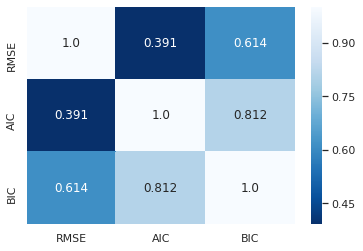In :
sns.jointplot(y='RMSE', x='BIC', data=test_results[['RMSE', 'BIC']].rank());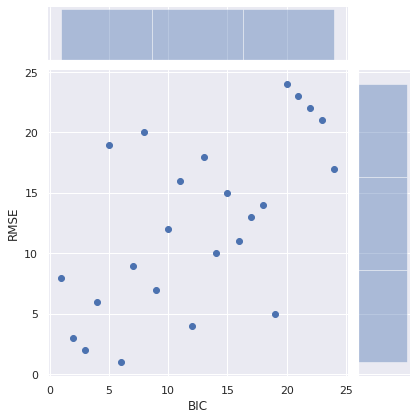In :
sns.heatmap(test_results.RMSE.unstack().mul(10), fmt='.3', annot=True, cmap='Blues_r')
b, t = plt.ylim()
b += 0.5
t -= 0.5
plt.ylim(b, t)
plt.show()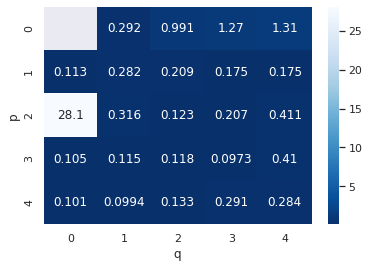We also aim to minimize BIC:

In :
sns.heatmap(test_results.BIC.unstack(), fmt='.2f', annot=True, cmap='Blues_r')
b, t = plt.ylim()
b += 0.5
t -= 0.5
plt.ylim(b, t)
plt.show()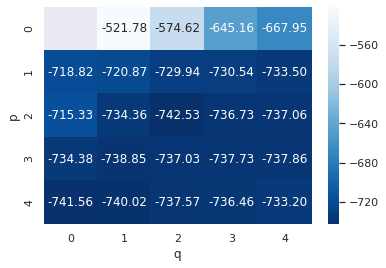### Estimating the best ARMA Model¶

ARMA(0,4) and ARMA(2,2) are close, with a slight edge to ARMA(2,2).

In :
# Just an ARMA(p,q) model
model = tsa.SARIMAX(endog=industrial_production_log_diff, order=(2, 0, 2))

results = model.fit()

print(results.summary())

                               SARIMAX Results
==============================================================================
Dep. Variable:                 IPGMFN   No. Observations:                  374
Model:               SARIMAX(2, 0, 2)   Log Likelihood                1190.533
Date:                Fri, 10 Apr 2020   AIC                          -2371.067
Time:                        02:14:37   BIC                          -2351.446
Sample:                    01-01-1989   HQIC                         -2363.276
- 02-01-2020
Covariance Type:                  opg
==============================================================================
coef    std err          z      P>|z|      [0.025      0.975]
------------------------------------------------------------------------------
ar.L1          1.8105      0.042     42.813      0.000       1.728       1.893
ar.L2         -0.8325      0.041    -20.287      0.000      -0.913      -0.752
ma.L1         -0.9152      0.061    -15.101      0.000      -1.034      -0.796
ma.L2          0.3341      0.051      6.562      0.000       0.234       0.434
sigma2       9.94e-05   6.47e-06     15.367      0.000    8.67e-05       0.000
===================================================================================
Ljung-Box (Q):                      101.73   Jarque-Bera (JB):                 9.04
Prob(Q):                              0.00   Prob(JB):                         0.01
Heteroskedasticity (H):               0.61   Skew:                            -0.16
Prob(H) (two-sided):                  0.01   Kurtosis:                         3.69
===================================================================================

Warnings:
 Covariance matrix calculated using the outer product of gradients (complex-step).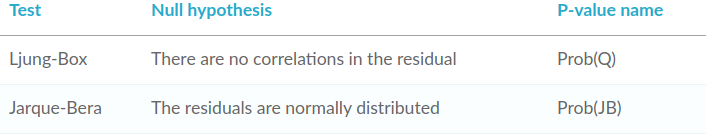### Plot diagnostics¶

If the model ts well the residuals will be white Gaussian noise.

In :
# Create the 4 diagostics plots
results.plot_diagnostics(figsize=(13, 8))
plt.show()